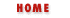### 7.3 Methods

A method is nothing more than a JavaScript function that is invoked through an object. Recall that functions are data values, and that there is nothing special about the name they are defined with--a function can be assigned to any variable, or even to any property of an object. If we have a function f, and an object o, then we can define a method named m with the following line:

```o.m = f;
```

Having defined the method m() of the object o, we invoke it like this:

```o.m();
```
Or, if m() expects two arguments, we might invoke it like this:

```o.m(x, x+2);
```

Invoking o.m() this way is the same as calling f(), except for one point: when the function is invoked as a method, through the object o, the this keyword will refer to that object within the body of the method. When the same function object is invoked directly as f(), the this keyword will not contain a meaningful value.

 As you may have discovered by now, variables in client-side JavaScript are all implicitly properties of the current Window object, so invoking f() is equivalent to invoking window.f(): the this keyword in both these cases refers to the current window. (See Chapter 11, Windows and the JavaScript Name Space, for an extended discussion of this somewhat odd aspect of JavaScript.)

This discussion of the this keyword should begin to make it clear why we use methods at all. Any function that is used as a method is effectively passed a third argument--the object through which it is invoked. Typically, a method performs some sort of operation on that object, and the method invocation syntax is a particularly elegant way to express the fact that a function is operating on an object. Compare the following two lines of code:

```o.m(x,y);
f(o,x,y);
```
The hypothetical method m() and function f() may perform exactly the same operation on the object o, but the method invocation syntax more clearly indicates the idea that it is the object o that is the primary focus or target of the operation.

The typical usage of methods is more clearly illustrated through an example. Example 7.2 returns to the Rectangle objects of Example 7.1 and how a method that operates on Rectangle objects can be defined and invoked.

#### Example 7.2: Defining and Invoking a Method

```// This is a function. It uses the this keyword, so
// it doesn't make sense to invoke this function by itself; it
// needs instead be made a method of some object, some object that has
// "width" and "height" properties defined.
function compute_area()
{
return this.width * this.height;
}
// Create a new Rectangle object, using the constructor defined earlier
var rect = new Rectangle(8.5, 11);
// Define a method by assigning the function to a property of the object
rect.area = compute_area;
// Invoke the new method like this:
a = rect.area();    // a = 8.5*11 = 93.5
```

There is a shortcoming that is evident in Example 7.2: before you can invoke the area() method for the rect object, you must assign that method to a property of the object. While we can invoke the area() method on the particular object named rect, we can't invoke it on any other Rectangle objects without first assigning the method to them. This quickly becomes tedious. Example 7.3 defines some additional Rectangle methods and shows how they can automatically be assigned to all Rectangle objects with a constructor function.

#### Example 7.3: Defining Methods in a Constructor

```// First, define some functions that will be used as methods
function Rectangle_area() { return this.width * this.height; }
function Rectangle_perimeter() { return 2*this.width + 2*this.height; }
function Rectangle_set_size(w,h) { this.width = w; this.height = h; }
function Rectangle_enlarge() { this.width *= 2; this.height *= 2; }
function Rectangle_shrink() { this.width /= 2; this.height /= 2; }
// Then define a constructor method for our Rectangle objects.
// The constructor initializes properties, and also assigns methods.
function Rectangle(w, h)
{
// initialize object properties
this.width = w;
this.height = h;
// define methods for the object
this.area = Rectangle_area;
this.perimeter = Rectangle_perimeter;
this.set_size = Rectangle_set_size;
this.enlarge = Rectangle_enlarge;
this.shrink = Rectangle_shrink;
}
// Now, when we create a rectangle, we can immediately invoke methods on it:
r = new Rectangle(2,2);
a = r.area();
r.enlarge();
p = r.perimeter();
```Creating New Objects with ConstructorsObject Prototypes